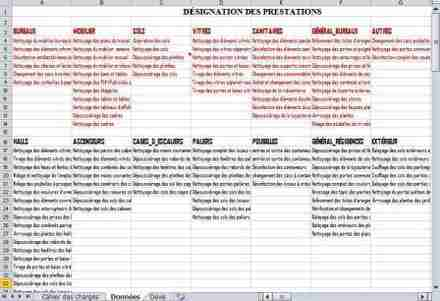# Excel - Specifications and limitations of SheetsThis tips aims to provide some information on the rationale of values ??(specifications and limits) apparently arbitrary under Excel:

Why is there a limitation of 256 displayable characters in a cell and not 250 or 300?

Why 16,384 columns in Excel 2007, and not 16,000 or 17,000?

Situation

"I'm faced with a limitation of 65,536 rows under Excel, but I do not understand the reasons for this limitation.. Could someone explain the logic behind?

Explanations

A byte consists of 8 bits (binary digit: 0 or 1) and can be represented as 2 ^ 8 = 256 different values. Hence the restriction

256 ASCII codes

256 displayable characters in an Excel cell,

256 columns in Excel 2003, etc..

Other limitations:

With 2 bytes, or 16 bits, you can define 2 ^ 16 = 65536 values, number of rows in Excel 2003.

With 14 bits, you can define 2 ^ 14 = 16384 values. This is the number of columns in Excel 2007.

With 20 bits, you can define 2 ^ 20 = 1048576 values. This is the number of rows in Excel 2007.

In the Excel 2007 Help Topics go to the "Specifications and limits for spreadsheets and workbooks" chapter:

You shall notice that that most of these limitations are based on power of 2:

2 ^ 4 = 16 layers and line styles

2 ^ 5 = 32 styles, fields in a form

2 ^ 9 = 512 fonts for workbook

2 ^ 10 = 1024 page breaks

2 ^ 15 = 32767 characters stored in a cell.

It should be noted that Excel 2010 specifications are the same as the ones used for Excel 2007.Next Post